# Surface Area And Volume Examples

The world we live in is three dimensional in nature. Every object can be measured in terms of length, breadth and height. For example, the house that we live in has dimensions. Surface area is the outer part of the figure whereas volume is the inner part. This article contains solved examples on surface area and volume.

## Surface Area And Volume Definitions

The complete area of the surface of a three dimensional solid can be termed as surface area. It is denoted by “S” and measured in square units.

The quantity of a three dimensional solid or the space taken by it is called volume. Volume is denoted by “V” and measured in cubic units.

## Surface Area And Volume Formulae

To find the surface area and volume of the solids individually or combined together, the table can be used for reference.

 Name Diagram of solid Lateral or Curved Surface Area Total Surface Area Volume Length of the diagonal and Explanation of terms Cube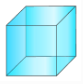4l2 6l2 l3 √3 l = edge of the cube Cuboid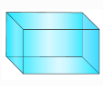2h(l +b) 2(lb + bh + hl) lbh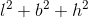l = length b = breadth h = height Cylinder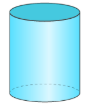2πrh 2πr2 + 2πh = 2πr(r + h) πr2h r = radius h = height Hollow cylinder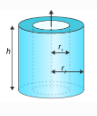2πh (R + r) 2πh (R + r) + 2πh (R2 – r2) – R = outer radius r = inner radius Cone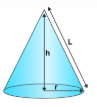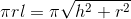πr2 + πrl = πr(r + l) 1/3 πr2h r = radius h = height l = slant height Frustum of a cone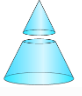πl(R + r) πR2+πr2+πl(R+r) ⅓ πh[R2+r2+Rr] – Sphere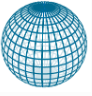4πr2 4πr2 4/3 πr3 r = radius Prism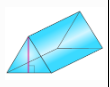Perimeter of base × height Lateral surface area + 2(Area of the end surface) Area of base × height – Pyramid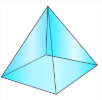1/2 (Perimeter of base) × slant height Lateral surface area + Area of the base 1/3 area of base × height –

## Surface Area And Volume Examples

Example 1: The radius and height of a cone are in the ratio 4 : 3. The area of the base is 154cm2. What is its curved surface area?

Solution:

$\pi[r^2]=154\\ r=7cm\\ \frac{r}{h}=\frac{7}{3}\\ \frac{7}{h}=\frac{4}{3}\\ h=\frac{21}{4}\\ Curved\ surface \ area=\pi[rl]\\ =\pi[r]\sqrt{r^2+h^2}\\ =[22/7]*7*\sqrt{7^2+[\frac{21}{4}]^2}\\ =192.25 cm^2$

Example 2: The percentage decrease in the surface area of the cube, when each side decreased to 0.5 times the original length is ____________.

Solution:

The surface area of the cube = 6a2

The new surface area of the cube = $6*[\frac{a}{2}]^2=\frac{6a^2}{4}$

Percent change = $[\frac{6a^2-\frac{6a^2}{4}}{6a^2}]*100$ $\frac{18a^2*100}{4*6a^2}$ $=75%$

Example 3: A circus tent is bent in the form of a cone over a cylinder. The diameter of the base is 9m, the height of the cylindrical part is 4.8m and the total height of the tent is 10.8m. What is the amount of canvas required for the tent?

Solution:

The surface area of the conical part

= $\pi r \times \sqrt{r^2+(h_1)^2}$

= $\pi \times 4.5 \times \sqrt{[4.5]^2+6^2}$

= $\frac{22}{7} \times 4.5 \times 7.5$

The surface area of the cylindrical part = $2\pi*rh{_2}$

Total surface area = $[\pi]r(\sqrt{r^2+[h_1]^2+2h_2})=241.84sq\ m$

Example 4: A toy of the shape of a cone over a hemisphere. The radius of the hemisphere is 3.5cm. The total height of the toy is 15.5 cm. Find the total area of the toy.

Solution:

The top is made up of two shapes, one is a hemisphere of radius r=3.5cm and the other cone of height h= 15.5 – 3.5 = 12cm

The total area of the top = $2\pi*(3.5)^2+[\pi]3.5*\sqrt{3.5^2+12^2}=214.5 \ sq\ cm$

Example 5: The dimensions of the cuboid are in the ratio of 1 : 2 : 3 and its total surface area is 88 cm2. What is the lateral surface area?

Solution:

Let the length, breadth and height of the cuboid be l, 2l and 3l respectively.

Total surface area = 2{(l * 2l) + (2l * 3l) + (3l * l)} = 88 cm2

22l2 = 88

l = 2 cm

Hence l = 2 cm, 2l = 4 cm, 3l = 6 cm

Lateral surface area = 2(l * 3l) + (2l * 3l) = 18l2 = 72 cm2

Example 6: A sphere, a cylinder and a cone are of the same height and same radius, then the ratio of their curved surfaces are ______.

Solution:

Let the

Radius of the sphere be r

Height of the sphere = 2r

Height of the cylinder = 2r

Height of the cone = 2r

Hence ratio of the curved surface area is

$=4 \pi r^{2}:2 \pi r (2r):\pi r\sqrt{r^{2}+(2r)^{2}}\\ =4 \pi r^{2}:4 \pi r^{2}:\pi r^{2}\sqrt{5}\\ =4:4:\sqrt{5}$

Example 7: Each side of a cube increases by 50%. Then the surface area of the cube increases by ______.

Solution:

Let each side of the cube be a.

After increment, each sides of the cube = $a+\frac{50a}{500}$

Surface area of the cube = $a^{2}$

Surface area, after increment $=6(\frac{a*3}{2})^{2}\\ =6a^{2}\times \frac{9}{4}\\$

$\text \ Increment \ in \ surface \ area=[\frac{\text \ Increment\ -\ \text \ Original}{\text \ Original}\times 100] \%\\ =[\frac{6a^{2}\times \frac{9}{4}-6a^{2}}{6a^{2}}\times 100] \% \\ =[\frac{5}{4}\times 100] \%\\ =125 \%\\$

Example 8: The level of water of a swimming pool is rectangular of which length is 32 metres and width is 9.5 metres and the depth of water at one end is $1\frac{1}{2}$ metres which increases to $4\frac{1}{2}$ metres at other end. Find the volume of water in the swimming pool.

Solution:

The cross-section of swimming pool is like a trapezium, of which parallel sides are $4\frac{1}{2}$ metres and $1\frac{1}{2}$ metres and vertical perpendicular is 32 metres.

Therefore the area of cross-section $=\frac{1}{2}\times 16\times \left( 4\frac{1}{2}+1\frac{1}{2} \right)=48$ sq.m.

Hence the volume of water in the swimming pool = area of the cross-section $\times$ length $=48\times 9.5=456$ cubic metres.

Example 9: The length of cold storage is twice its breadth. The height is 3 metres and the area of its four walls (including doors) is 180 in. Find its volume?

Solution:

Let the length, breadth and height of the cold storage be l metres, b metres and h metres respectively.

Then, l = 2b (given) and h = 3 metres.

Now, area of four walls = 108

⇒ 2 (l + b) h = 108

⇒ 2 (2b + b) × 3 = 108

⇒ 18b = 108

⇒ b = 6 metres

∴l = 2b

⇒ l = 12 metres

Hence, volume of the cold storage = (lbh) m3 = (12 × 6 × 3) m3 = 216 m3.

Example 10: A cone and a cylinder have the same curved surface area and base area. If the height of the cylinder is 3m, then find the slant height of the cone.

Solution:

Cone and cylinder have the same base area. It implies that they have the same radius.

Curved surface area of cone = Curved surface area of cylinder

πrl = 2πrh

l = 2h

l = 2 * 3

l = 6

Test your Knowledge on surface area and volume